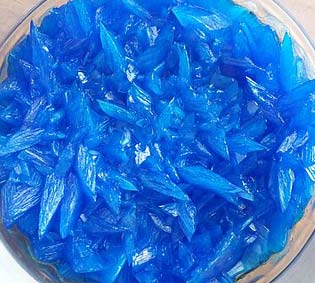Edexcel triple science only (not for double science students).

1. Which statement about solubility is true?
• A.   A saturated solution is a solution which is able to dissolve more solid
• B.   The solubility of most salts increases with temperature
• C.   A solubility of ‘20g per 100g of water’ means 100cm3 of solution contains 20g of solid
• D.   All salts are soluble in water at room temperature

2. The graph shows the solubility in water of 4 solids at different temperatures:Which solid is the most soluble at 20°C?

3. Solubility curves for NaCl and KNO3 are shown below:Which statement about the solubility of KNO3 and NaCl in water is NOT true?

• A.   The solubility of KNO3 increases with temperature
• B.   At 50°C, NaCl is less soluble than KNO3
• C.   At 70°C, 80g of KNO3 would all dissolve in 100g of water
• D.   NaCl is much more soluble at 80°C than at 10°C

Q4-7:

The solubility curve for copper(II)sulfate in water is shown below:4. What will happen to a hot saturated solution of copper(II)sulfate as the temperature decreases?
• A.   The solvent will evaporate
• B.   The solution will turn from blue to colourless
• C.   Crystals will form
• D.   No visible change will occur
5. What is the maximum mass of copper(II)sulfate that will dissolve in 20g of water at 40°C?
• A.   59 g
• B.   28 g
• C.   11.8 g
• D.   5.6 g
6. 22g of copper(II)sulfate was dissolved in 50g of water at 80°C. The solution was slowly cooled. At what temperature would crystals begin to form?
• A.   64°C
• B.   62°C
• C.   32°C
• D.   28°C
7. A saturated solution of copper(II)sulfate was prepared by dissolving the solid in 200g of water at 80°C.

What mass of crystals would form if the solution was cooled to 40°C?• A.   28g
• B.   30g
• C.   56g
• D.   60g

Q8-9:
A student carried out an experiment to find the solubility of potassium chloride at 30°C. He produced a saturated solution of potassium chloride at 30°C and then heated a known mass of the saturated solution until all the water evaporated.
The student obtained the following results:

 Mass of saturated potassium chloride solution heated / g 45 Mass of solid potassium chloride obtained /g 12.2

8. The solubility of potassium chloride at 30°C in grams per 100g of water is ……
• A.    12.2
• B.    27.1
• C.    37.2
• D.    72.9
9. The reliability of the experiment could be improved by ……..
• A.   Repeating the experiment three times and averaging the results
• B.   Carrying out the experiment at a range of temperatures
• C.   Evaporating a larger mass of solution
• D.   Repeating the experiment with other potassium salts

10. There are three different methods for preparing pure, dry samples of a salt:

 1. Adding an excess insoluble solid to acid 2. Adding alkali to acid 3. Precipitation

Which method is most suitable to prepare each of the salts in the table below?

 Lead(II)sulfate Copper(II)sulfate ammonium sulfate A 3 2 1 B 1 2 3 C 2 1 3 D 3 1 2